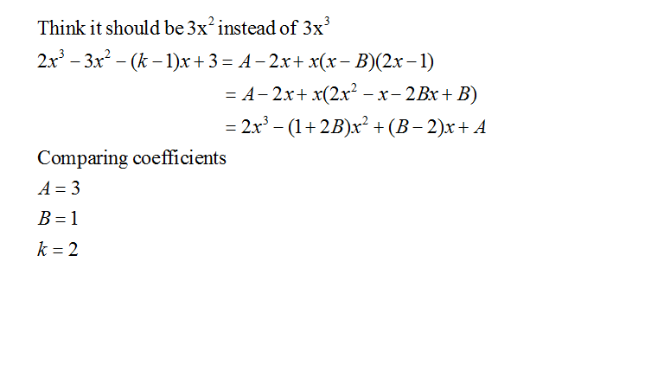# Question

It is given that 2x3-3x3-(k-1)x+3=A-2x+x(x-B)(2x-1). Find the values of A, B and k.Thanks again siglapmath,  I double check her worksheet its 3x

given answer is A=3 B=1 , k=2